## Overview

While music visualization in tabr focuses on leveraging LilyPond to make tablature, some diagrams can also be drawn directly in R using ggplot without any need to involve LilyPond. The plot_fretboard function makes standalone fretboard diagrams in R, independent of the LilyPond sheet music pipeline.

plot_fretboard is a highly specialized function that takes vector inputs for string numbers and fret numbers and maps the combination element-wise to produce a fretboard diagram. Note that plot_fretboard is a developmental function and the interface and arguments it provides may change.

The fretted notes are marked in the diagram using customary large circles.

plot_fretboard(string = 6:1, fret = c(0, 2, 2, 0, 0, 0))## Labels and tuning

The fretted notes can be labeled. labels can be an arbitrary vector corresponding to the string and fret numbers. For example, you can label each circle with the fingerings used to play chords or scales.

plot_fretboard(6:1, c(0, 2, 2, 0, 0, 0), c("G", "U", "I", "T", "A", "R"))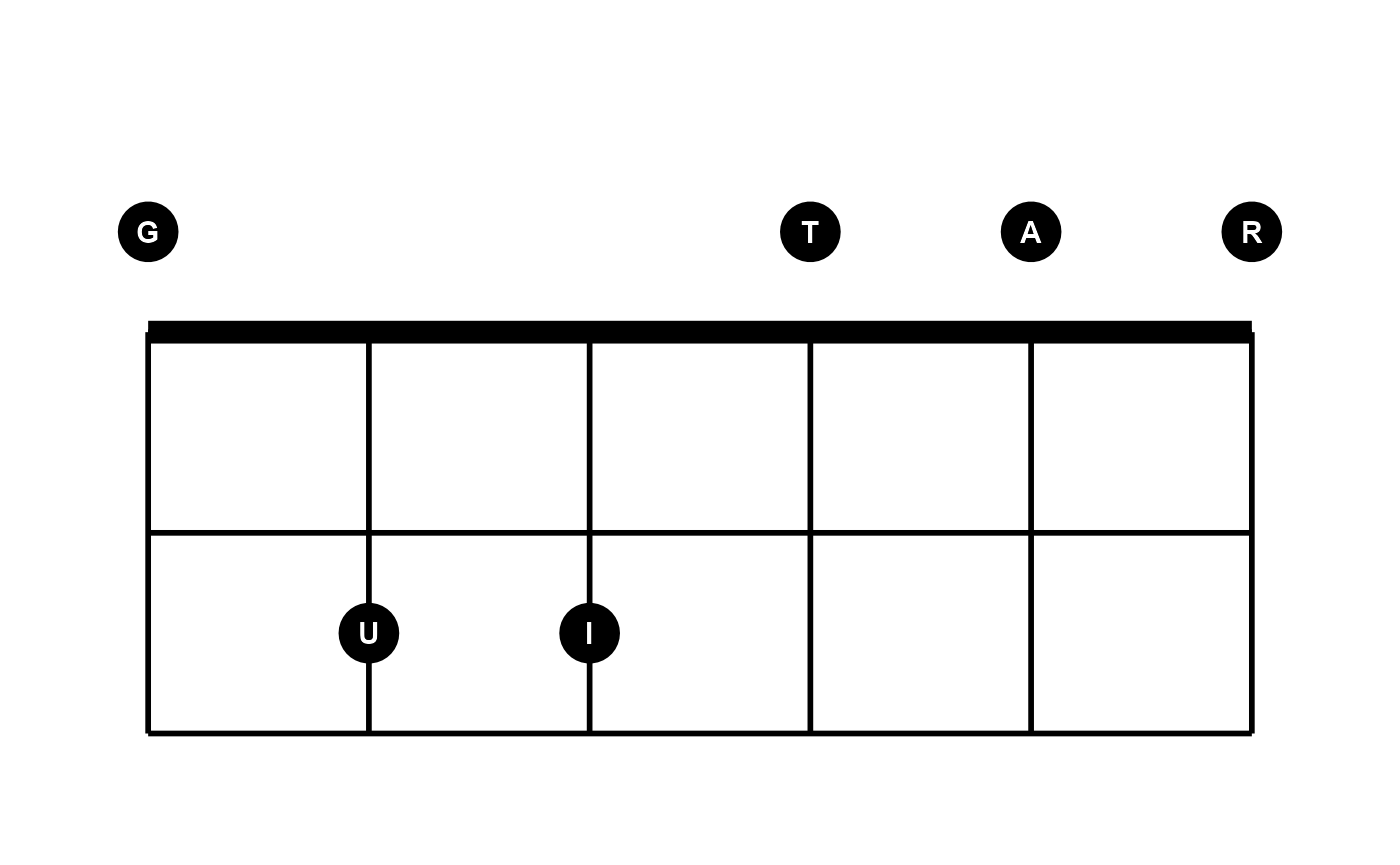If you set labels = "notes", this is a special setting that will label all points with their note names. This can be done automatically because providing string and fret numbers in conjunction with the tuning argument gives full information about the notes along the guitar neck. plot_fretboard transposes these internally. This means it will work automatically no matter what arbitrary tuning you set. Here is an example that also displays the tuning.

string <- c(6, 6, 6, 5, 5, 5, 4, 4, 4, 4, 4, 3, 3, 3, 2, 2, 2, 1, 1, 1)
fret <- c(2, 4, 5, 2, 4, 5, 2, 4, 6, 7, 9, 6, 7, 9, 7, 9, 10, 7, 9, 10)
plot_fretboard(string, fret, "notes", show_tuning = TRUE)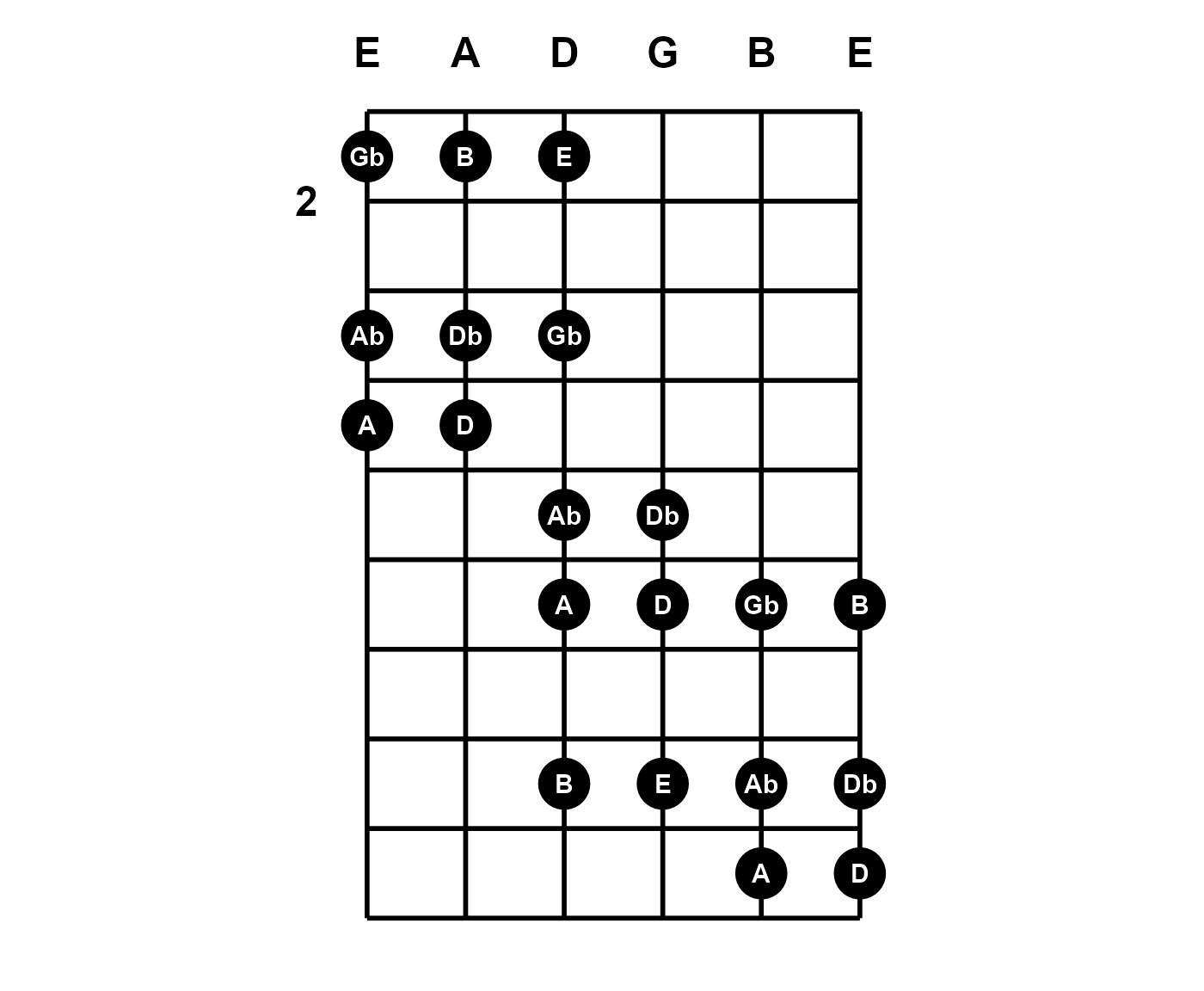When labels = "notes", accidentals are displayed as flats by default. Set accidentals = "sharp" to change.

plot_fretboard(string, fret, "notes", show_tuning = TRUE, accidentals =  "sharp")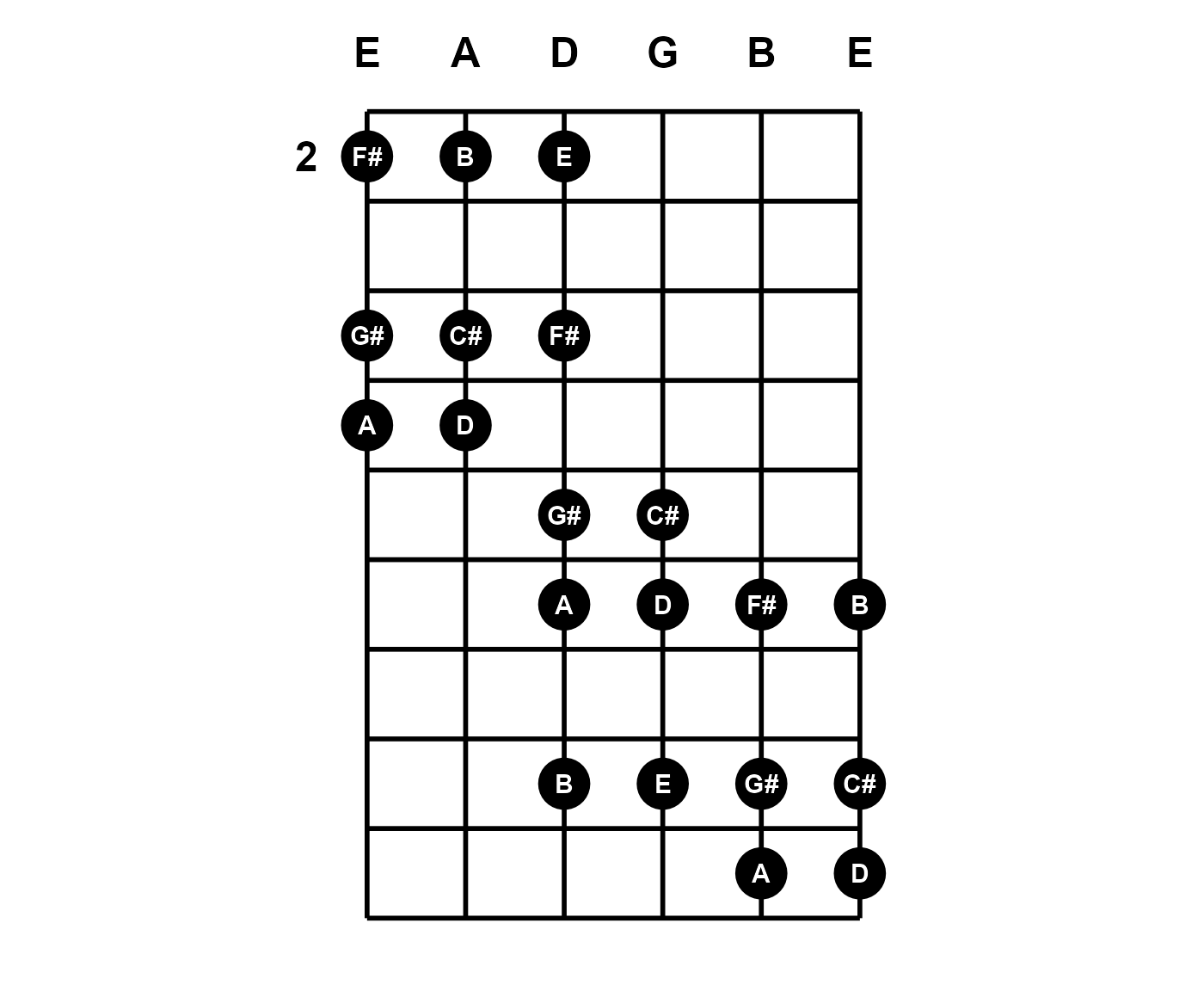## Limits

X and Y limits are expressed in terms of the number of instrument strings and the span of frets. You can override the fret range that is derived from fret:

plot_fretboard(string, fret, "notes", fret_range = c(0, 10), show_tuning = TRUE)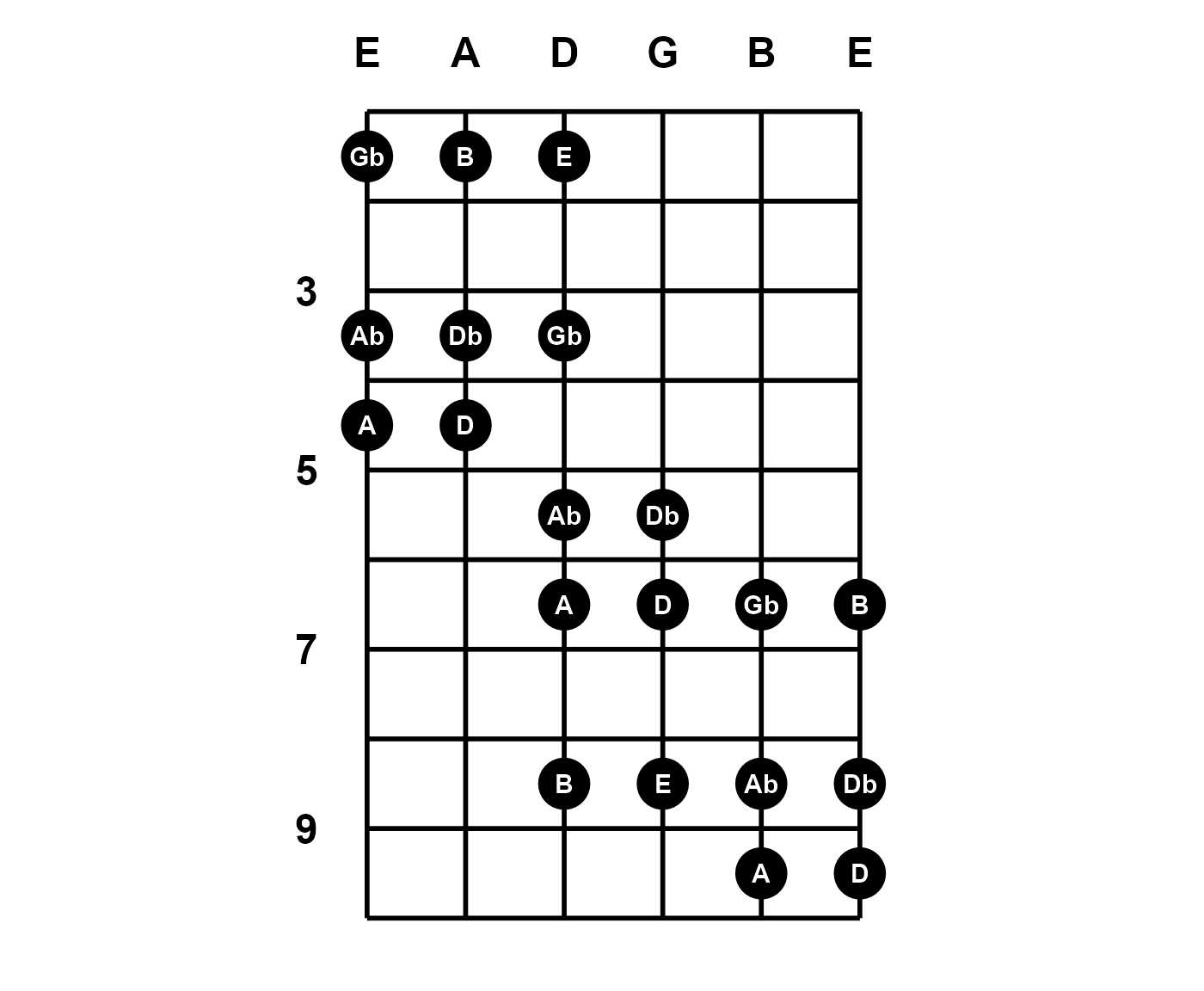The number of strings an instrument has is derived from tuning and this generalizes the fretboard diagram possibilities further. The tuning now specifies a seven-string guitar. One note has been added on string seven:

tuning <- "b1 e2 a2 d3 g3 b3 e4"
plot_fretboard(c(7, string), c(1, fret), "notes", fret_range = c(0, 10),
tuning = tuning, show_tuning = TRUE)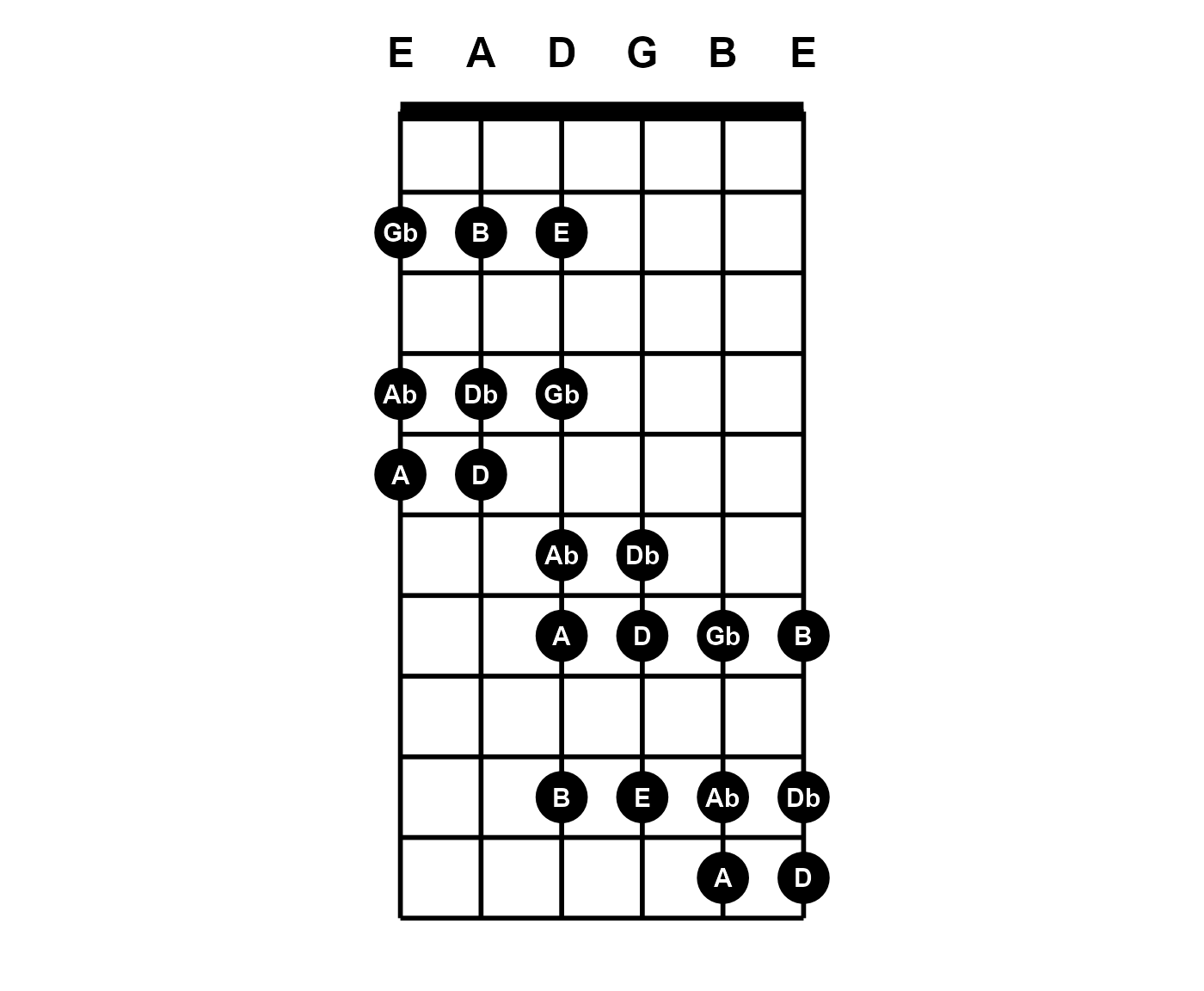## Color and faceting

The points (circles) can have border and fill color. The labels can also be colored separately. These arguments can be vectorized. Notice the open Am chord does not really have an open sixth string; it is muted. The zero is still given in order to specify where to notate, but the mute argument is given with a logical vector that indicates this entry is muted.

am_frets <- c(c(0, 0, 2, 2, 1, 0), c(5, 7, 7, 5, 5, 5))
am_strings <- c(6:1, 6:1)
mute <- c(TRUE, rep(FALSE, 11))

# colors
idx <- c(2, 2, 1, 1, 1, 2, rep(1, 6))
lab_col <- c("white", "black")[idx]
pt_fill <- c("firebrick1", "white")[idx]

plot_fretboard(am_strings, am_frets, "notes", mute,
label_color = lab_col, point_fill = pt_fill)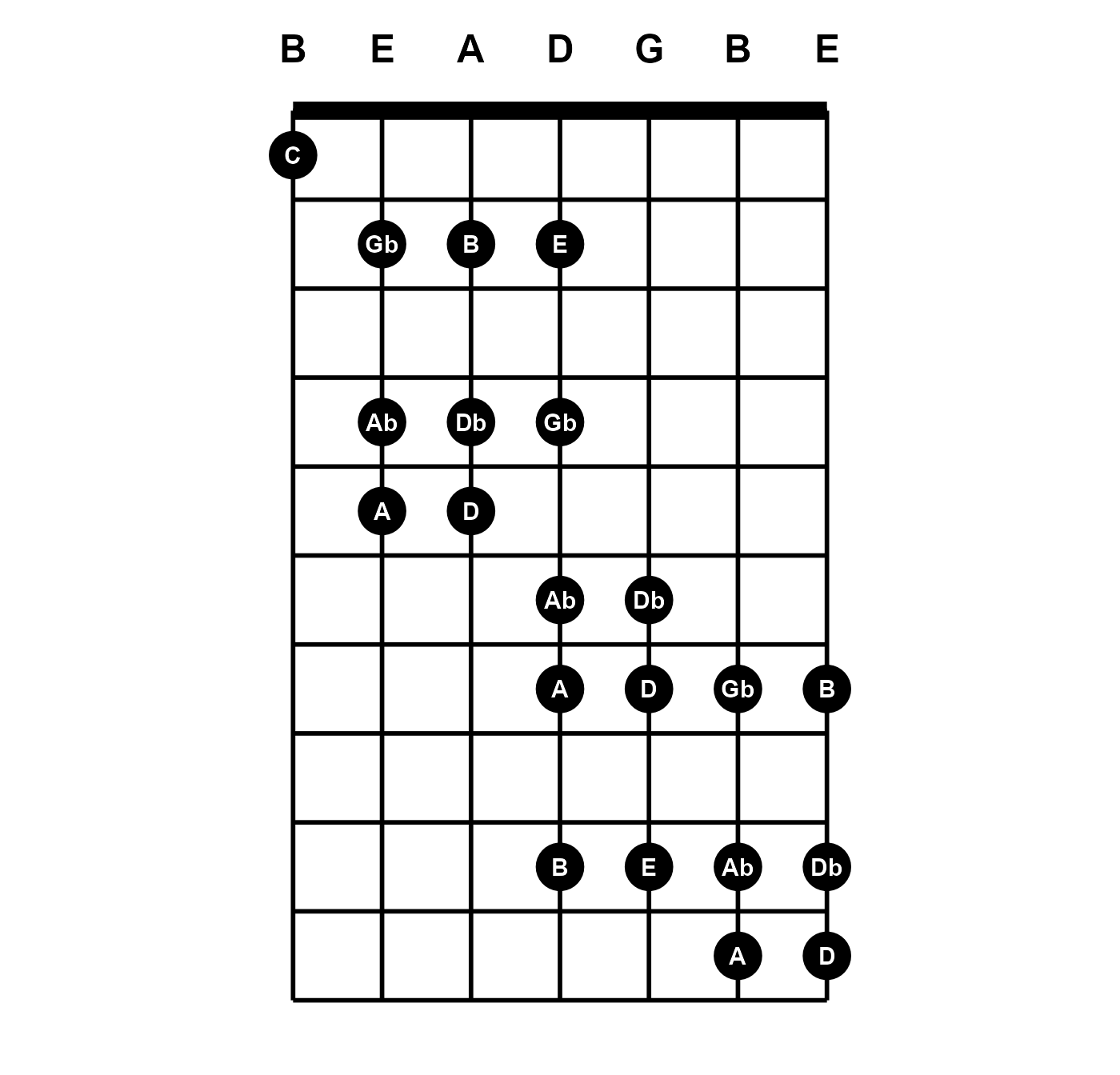group can also be used for faceting. However, faceting is still a problematic feature. It may work well enough in cases where the different diagrams span similar frets. The presence of muted notes can also cause issues when faceting. plot_fretboard works best for single-panel plots. Since the function returns a ggplot object, you can always make them separate plots and arrange in a grid layout rather than rely on within-plot faceting.

Note that plot_fretboard accepts character inputs like those used throughout tabr:

f <- "0 2 2 1 0 0 0 2 2 0 0 0"
s <- c(6:1, 6:1)
grp <- rep(c("Open E", "Open Em"), each = 6)

# colors
idx <- c(2, 1, 1, 1, 2, 2, 2, 1, 1, 2, 2, 2)
lab_col <- c("white", "black")[idx]
pt_fill <- c("firebrick1", "white")[idx]

plot_fretboard(s, f, "notes", group = grp, fret_range = c(0, 4),
label_color = lab_col, point_fill = pt_fill)## Orientation

The direction and handedness can also be changed. Diagrams can be vertical or horizontal as well as left- or right-handed.

Here, titles are added to the ggplot objects with ggtitle. Of course you can add onto ggplot objects returned by plot_fretboard, but you are limited in what you can add on and must be careful to avoid overriding properties of layers plot_fretboard has already specified.

library(ggplot2)
plot_fretboard(string, fret, "notes", label_color = "white", point_fill = "dodgerblue",
fret_range = c(0, 10), show_tuning = TRUE, horizontal = TRUE) +
ggtitle("Horizontal")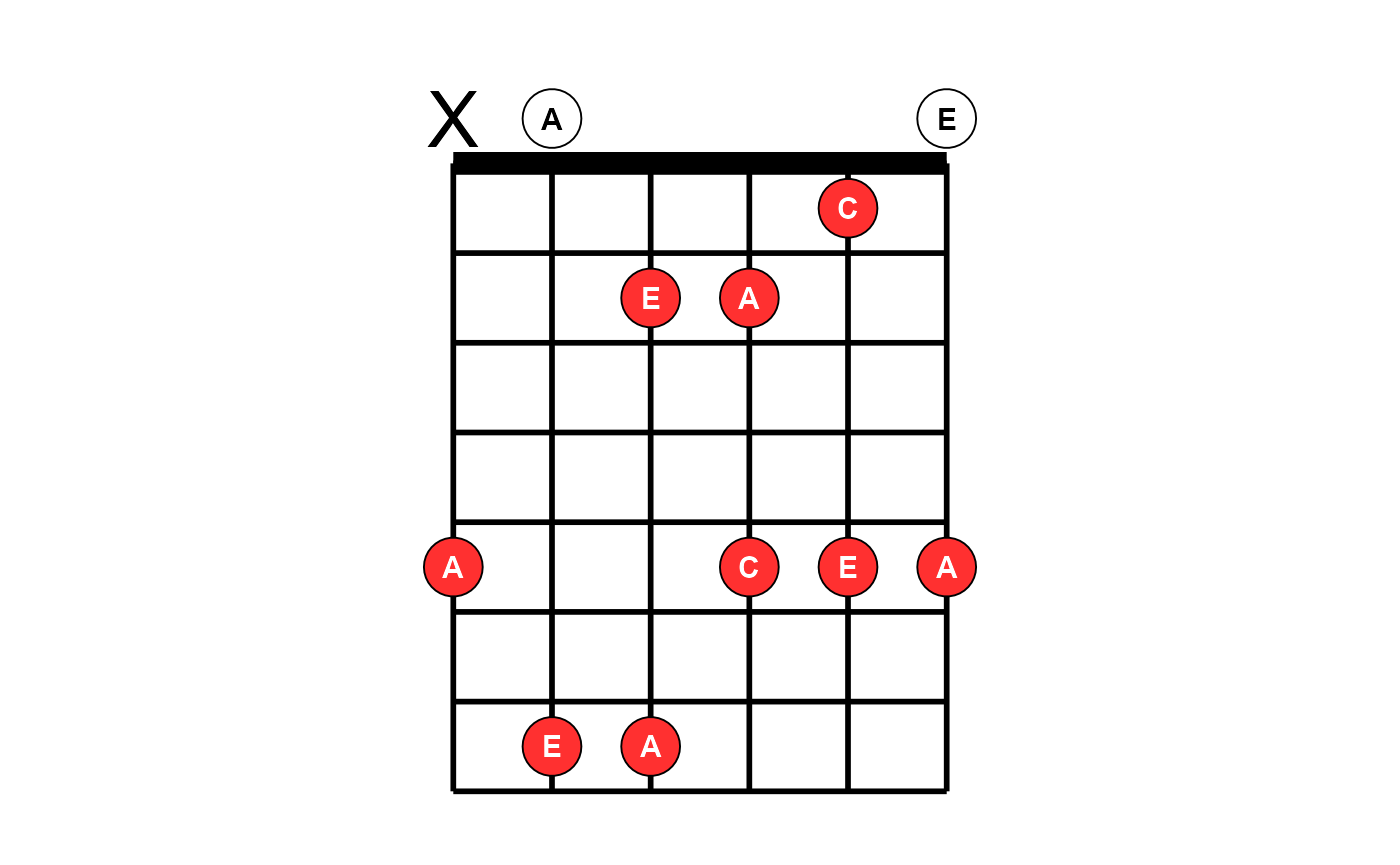plot_fretboard(string, fret, "notes", label_color = "white", point_fill = "dodgerblue",
fret_range = c(0, 10), show_tuning = TRUE, horizontal = TRUE, left_handed = TRUE) +
ggtitle("Horizontal and left-handed")## Chord diagrams

The previous examples show a mix of using plot_fretboard to make general fretboard diagrams that show scales, arpeggios and other patterns, as well as to produce chord diagrams of specific chords. It is easier to use plot_chord for the latter. It is a wrapper around plot_fretboard that takes a string representing a single chord in the simple fret format shown here.

idx <- c(1, 1, 2, 2, 2, 1)
fill <- c("white", "black")[idx]
lab_col <- c("black", "white")[idx]
plot_chord("xo221o", "notes", label_color = lab_col, point_fill = fill)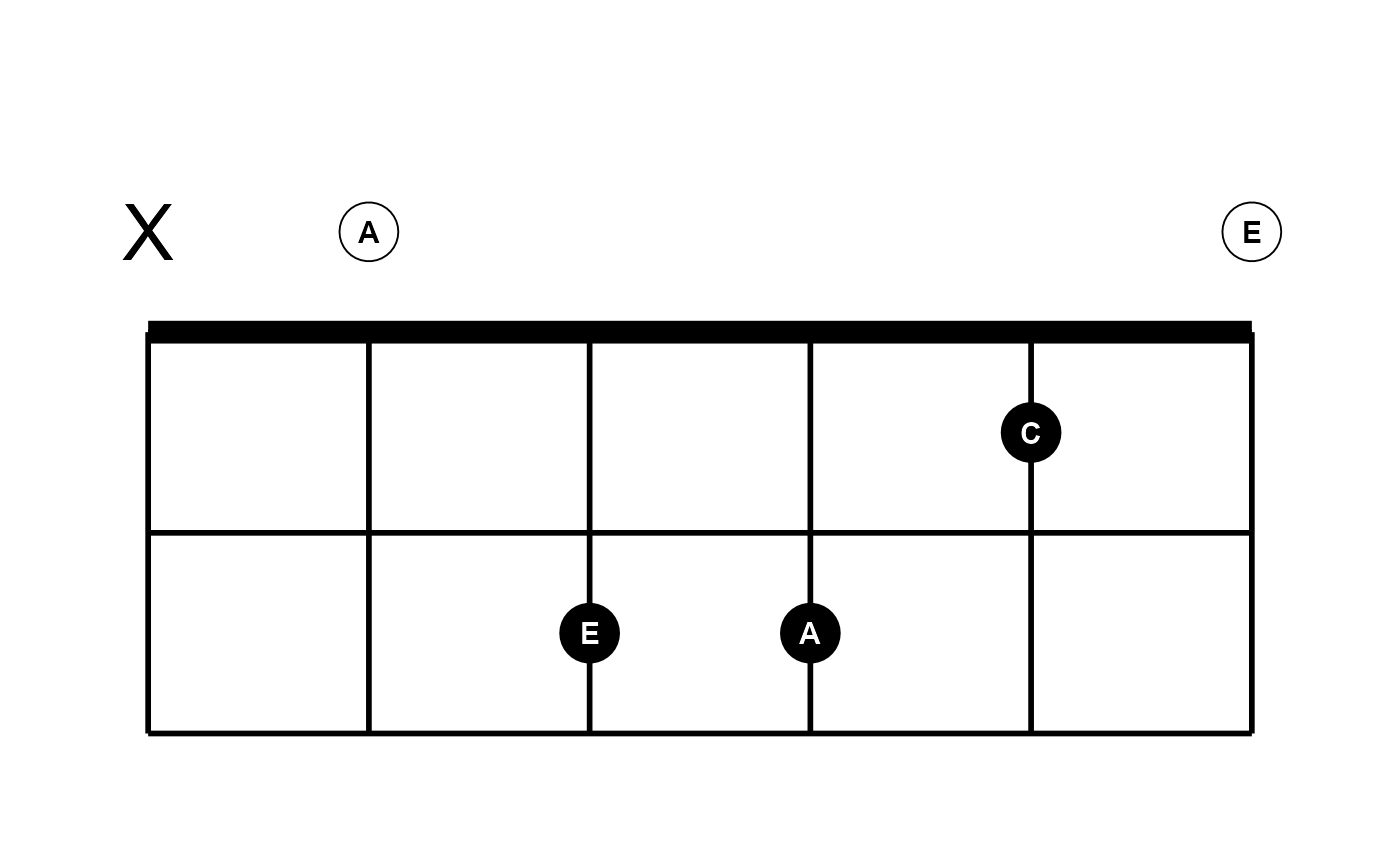Leading x is inferred if there are fewer fret values in the string than there are instrument strings, as inferred from the tuning argument. plot_chord takes all the same arguments as plot_fretboard except that it takes chord instead of string and fret and it does not use mute because muted notes are indicated with an x inside chord.

plot_chord("0231")Frets are assumed to be single-digit when provided as above. When any two-digit fret value occurs, you must provide the fret values as space- or semicolon-delimited. The latter is like LilyPond format, but a trailing semicolon is ignored if present.

plot_chord("10 12 13 11", fret_range = c(10, 14)) # same as "10;12;13;11"In an effort to leave some flexibility to the user, and while these plotting functions remain under development, plotting multiple chords as a chord chart is intentionally handled separately on a case by case basis. Here is an example using a little extra ggplot2, purrr and gridExtra. It also shows how you can tweak some layout settings to size and arrange elements nicely for your Rmd file.

library(ggplot2)
library(purrr)
library(gridExtra)

chords <- c("02210", "32010", "0232", "133211", "022100")
id <- c("Am", "C", "D", "F", "E")

g <- map2(chords, id, ~{
plot_chord(.x, "notes", point_size = 8, fret_range = c(0, 4), accidentals = "sharp", asp = 1.25) +
ggtitle(.y)
})

grid.arrange(grobs = g, nrow = 2)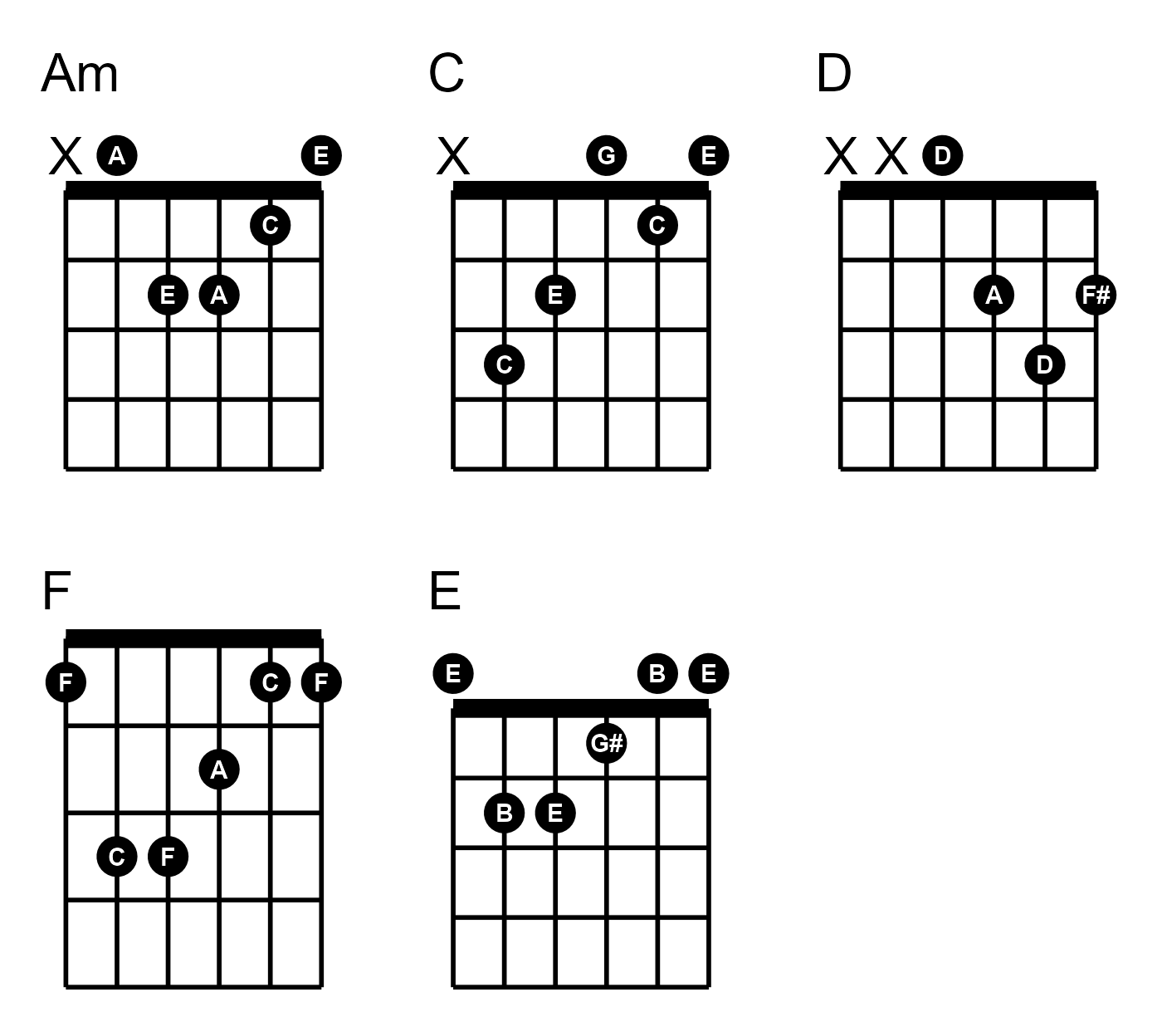You can use marrangeGrob instead of grid.arrange if you want to split a larger set of chord diagrams onto multiple pages.